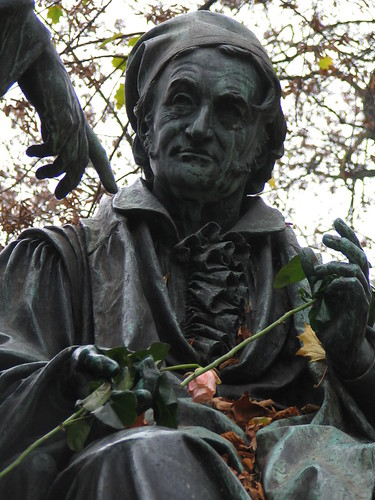## Gauss to Laplace transmutation interpretedFollowing my earlier post [induced by browsing X validated], on the strange property that the product of a Normal variate by an Exponential variate is a Laplace variate, I got contacted by Peng Ding from UC Berkeley, who showed me how to derive the result by a mere algebraic transform, related with the decomposition

(X+Y)(X-Y)=X²-Y² ~ 2XY

when X,Y are iid Normal N(0,1). Peng Ding and Joseph Blitzstein have now arXived a note detailing this derivation, along with another derivation using the moment generating function. As a coincidence, I also came across another interesting representation on X validated, namely that, when X and Y are Normal N(0,1) variates with correlation ρ,

XY ~ R(cos(πU)+ρ)

with R Exponential and U Uniform (0,1). As shown by the OP of that question, it is a direct consequence of the decomposition of (X+Y)(X-Y) and of the polar or Box-Muller representation. This does not lead to a standard distribution of course, but remains a nice representation of the product of two Normals.

### One Response to “Gauss to Laplace transmutation interpreted”

1.xi'an Says:

Here is another X validated entry about a product XY of a Beta B(1,K-1) X by an Chi-square χ²(2K) Y, ending up as an Exponential E(1/2)…. Easier but nice!

This site uses Akismet to reduce spam. Learn how your comment data is processed.